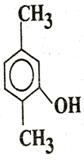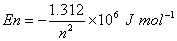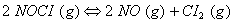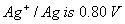Wednesday 25th November 2020

CBSE Guess > Papers > Question Papers > Class XII > 2004 > Chemistry > Compartment Delhi Set-II

CHEMISTRY (Set II—Compartmet Delhi)

Except for the following questions, all the remaining questions have been asked in Set I.

Q. 2. What type of crystal defect is produced when sodium chloride is doped with MgCl2 ? 1

Q. 3. Why is it that the elevation in boiling point of water is not the same in the following solutions? 1
0.1 molar NaCl solution and 0.1 molar sugar solution

Q. 4. In some cases it is found that a large number of colliding molecules have energy more than threshold value, yet the reaction is slow. Why? 1

Q. 5. Write IUPAC name ofQ. 6. The quantized energy of an electron in hydrogen atom for nth level is given byCalculate the energy required to remove the electron completely from an excited hydrogen atom when its quantized level, n =3.
(NA = 6.02 x 1023 mol-1) 2

Q. 7. What is the value of equilibrium constant for the following reaction at 400 K?Q. 11. (a) How does vulcanization change the properties of natural rubber?
(b) Why are the numbers 66 and 6 put in the name of nylon 66 and nylon 6? 2

Q. 13. (a) Classify the following processes as reversible or irreversible:
(i) Dissolution of sodium chloride
(ii) Evaporation of water at 373 K and 1 atm. Pressure
(iii) Mixing of two gases by diffusion
(iv) Melting of ice without rise in temperature
(b) When an ideal gas expands in vacuum, there is neither absorption nor evolution of heat. Why? 3

Q. 23. (a) Calculate the electrode potential of silver electrode dipped in 0.1 M solution of silver nitrate at 298 K assuming AgNO3 to be completely dissociated. The standard electrode potential ofat 298 K.
(b) At what concentration of silver ions will this electrode have a potential of 0.0V?

Q. 25. (a) Explain the cause and two consequences of lanthanoid contraction
(b) Give balanced chemical equations of two reactions in which KMnO4 acts as an oxidizing agent in the acidic medium. 3, 2

 Chemistry 2004 Question Papers Class XII Delhi Outside Delhi Compartment Delhi Compartment Outside DelhiSet ISet ISet ISet ISet IISet IISet IISet IISet IIISet III

CBSE 2004 Question Papers Class XII